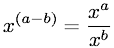Algebra > Exponents/PowersShowing results 1 to 9 of 9, on page 1 of 1
 DESCRIPTION EQUATION Addition of Exponents RuleDefinition of Square RootDistributive Property of Exponents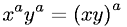Exponent Equal to One Rule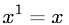Exponent Equal to Zero RuleFractional Exponent to Fractional Root Relationship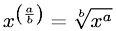Negative Exponent Definition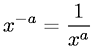Power Rule of ExponentsSubtraction of Exponents Rule